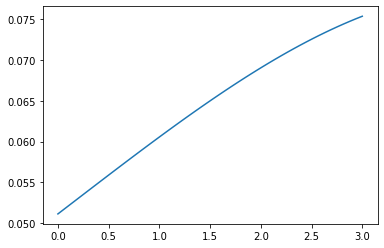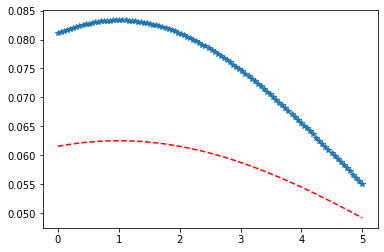Python – Logistic Distribution in Statistics

• Last Updated : 10 Jan, 2020

scipy.stats.logistic() is a logistic (or Sech-squared) continuous random variable. It is inherited from the of generic methods as an instance of the rv_continuous class. It completes the methods with details specific for this particular distribution.

Parameters :

q : lower and upper tail probability
x : quantiles
loc : [optional]location parameter. Default = 0
scale : [optional]scale parameter. Default = 1
size : [tuple of ints, optional] shape or random variates.
moments : [optional] composed of letters [‘mvsk’]; ‘m’ = mean, ‘v’ = variance, ‘s’ = Fisher’s skew and ‘k’ = Fisher’s kurtosis. (default = ‘mv’).

Results : Logistic (or Sech-squared) continuous random variable

Code #1 : Creating logistic (or Sech-squared) continuous random variable

 # importing library  from scipy.stats import logistic      numargs = logistic.numargs a, b = 4.32, 3.18rv = logistic(a, b)     print ("RV : \n", rv)

Output :

RV :
scipy.stats._distn_infrastructure.rv_frozen object at 0x000002A9D691AF48

Code #2 : logistic (or Sech-squared) continuous variates and probability distribution

 import numpy as np quantile = np.arange (0.03, 2, 0.21)   # Random Variates R = logistic.rvs(a, b) print ("Random Variates : \n", R)   # PDF R = logistic.pdf(a, b, quantile) print ("\nProbability Distribution : \n", R)

Output :

Random Variates :
2.655712569915262

Probability Distribution :
[3.09335001e-48 2.86886306e-04 2.07194337e-02 7.76003172e-02
1.34094156e-01 1.71154982e-01 1.89845362e-01 1.96114490e-01
1.95030508e-01 1.89935182e-01]

Code #3 : Graphical Representation.

 import numpy as np import matplotlib.pyplot as plt      distribution = np.linspace(0, np.minimum(rv.dist.b, 3)) print("Distribution : \n", distribution)      plot = plt.plot(distribution, rv.pdf(distribution))

Output :

Distribution :
[0.         0.06122449 0.12244898 0.18367347 0.24489796 0.30612245
0.36734694 0.42857143 0.48979592 0.55102041 0.6122449  0.67346939
0.73469388 0.79591837 0.85714286 0.91836735 0.97959184 1.04081633
1.10204082 1.16326531 1.2244898  1.28571429 1.34693878 1.40816327
1.46938776 1.53061224 1.59183673 1.65306122 1.71428571 1.7755102
1.83673469 1.89795918 1.95918367 2.02040816 2.08163265 2.14285714
2.20408163 2.26530612 2.32653061 2.3877551  2.44897959 2.51020408
2.57142857 2.63265306 2.69387755 2.75510204 2.81632653 2.87755102
2.93877551 3.        ]Code #4 : Varying Positional Arguments

 import matplotlib.pyplot as plt import numpy as np      x = np.linspace(0, 5, 100)      # Varying positional arguments y1 = logistic .pdf(x, 1, 3) y2 = logistic .pdf(x, 1, 4) plt.plot(x, y1, "*", x, y2, "r--")

Output :My Personal Notes arrow_drop_up